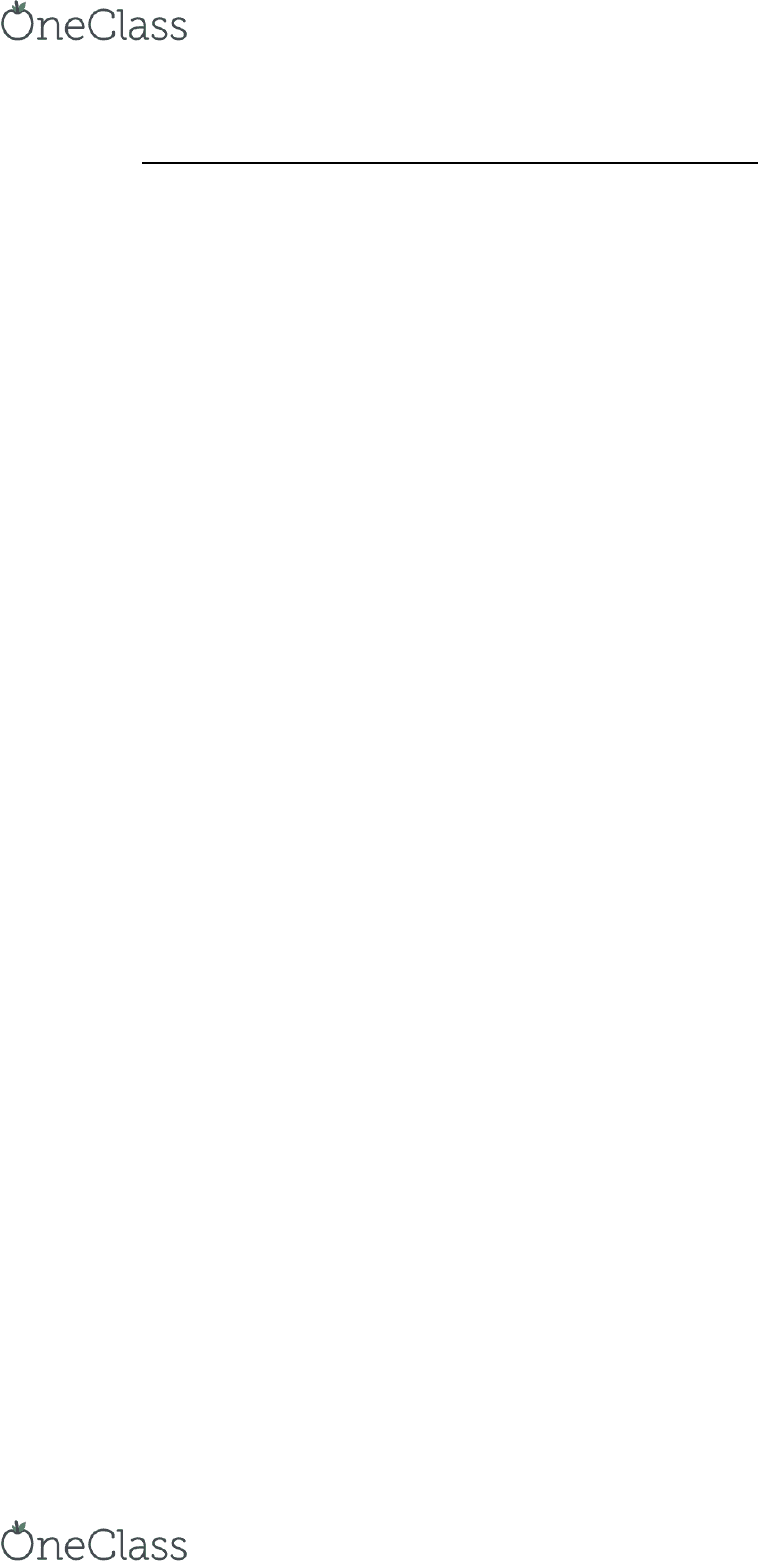Textbook Notes (270,000)
CA (160,000)
Trent (1,000)
Chapter 11

ADMN 3221H Chapter Notes - Chapter 11: Book Value, Income Statement, Impaired Asset

Department
Course Code
Professor
Chapter
11

This preview shows page 1. to view the full 5 pages of the document.Chapter 11 Depreciation, Impairment, and Disposition
Depreciation
Depreciation (and amortization more broadly) is a means of cost allocation
It is not a method of valuation
Depreciation involves:
o Allocating the depreciable amount of property, plant, and equipment
over the periods expected to benefit from the use of the assets
This allocation is generally recognized as Depreciation Expense
Factors in the Depreciation Process
What asset components are depreciated separately?
What is the asset’s depreciable amount?
Over what period is the asset depreciated?
What pattern best reflects how the asset’s economic benefits are used up?
Asset Components
Each significant part of a PP&E asset should be identified and depreciated as
a separate component
Multiple components may be grouped for calculating depreciation if they
have same useful lives and depreciation methods
Parts of each PP&E asset that are not individually significant can be grouped
and depreciated as a single component
Application of components for the purpose of depreciation is required by
both ASPE and IFRS. However, IFRS is more detailed and strict.
Depreciable Amount
Depreciable amount is initially calculated as:
o Original cost of the asset
o Less estimated residual value (or salvage value)
IFRS does not permit the use of salvage value
Residual value is the net amount expected to be received for the asset today
if it were of the age, and in the condition expected at the end of its useful life
Salvage value is the asset’s estimated net realizable value at the end of the
asset’s life
Residual value should be reviewed regularly (at least annually under IFRS)
Depreciation continues as long as residual value is lower than asset’s
carrying amount
Depreciation Period
Depreciation begins when the asset is available for use
Depreciation ends when the asset is derecognized or classified as held for
sale.
An asset’s useful life and physical life are not the same expressed in time or
units)
find more resources at oneclass.com
find more resources at oneclass.com

Unlock to view full version

Only page 1 are available for preview. Some parts have been intentionally blurred.Useful life is sometimes referred to as the economic lifethe period of time
over which the asset will produce revenue for the company
Factors affecting useful life are:
o Economic factors (e.g. obsolescence)
o Physical factors (e.g. wear and tear)
o Legal life (e.g. expiration of contract)
Depreciation Methods of Allocation and Calculation
Depreciation method determines the systematic allocation of the depreciable
amount over the asset’s useful life
Depreciation should reflect the pattern of benefits expected from the use of
the asset
Additional considerations for choosing a particular depreciation method
include simplicity, cost, as well as perceived economic consequences
Depreciation method affects:
o The statement of financial position
o The income statement
o The ratios (e.g. return on assets, etc.)
The three major systematic methods:
o Straight-line method
o Diminishing balance method
o Unit of production (or activity) method
Straight-Line Method
Most widely used
(Cost residual value)/estimated useful life
Based on two assumptions:
o Asset delivers equal economic benefits each year
o Maintenance expense is about same each period
Distorts rate of return analysis
Diminishing Balance Method
Uses a depreciation rate that stays constant throughout the asset’s life,
assuming there is no change in estimate
Depreciation rate is expressed as a percentage and is called the declining-
balance rate
Rate is applied each year tot he net book value (cost less accumulated
depreciation and any accumulated impairment losses)
Applies appropriate rate to the asset’s carrying amount at beginning of each
period
When residual value is reached, the asset is no longer depreciated
Units of Production Method
Calculates depreciation according to usage or productivity instead of the
passage of time
find more resources at oneclass.com
find more resources at oneclass.com